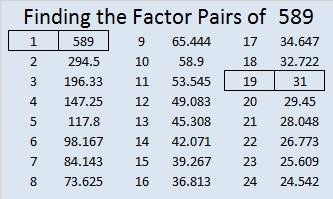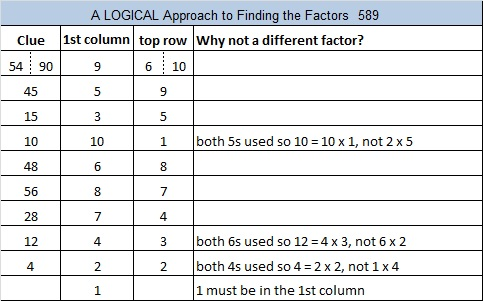# 589 and Level 4

589 is the sum of the three prime numbers from 193 to 199. It should be pretty obvious what that middle prime number is.Print the puzzles or type the solution on this excel file: 10 Factors 2015-08-17

—————————————————————————————————

• 589 is a composite number.
• Prime factorization: 589 = 19 x 31
• The exponents in the prime factorization are 1 and 1. Adding one to each and multiplying we get (1 + 1)(1 + 1) = 2 x 2 = 4. Therefore 589 has exactly 4 factors.
• Factors of 589: 1, 19, 31, 589
• Factor pairs: 589 = 1 x 589 or 19 x 31
• 589 has no square factors that allow its square root to be simplified. √589 ≈ 24.269322—————————————————————————————————Advertisements